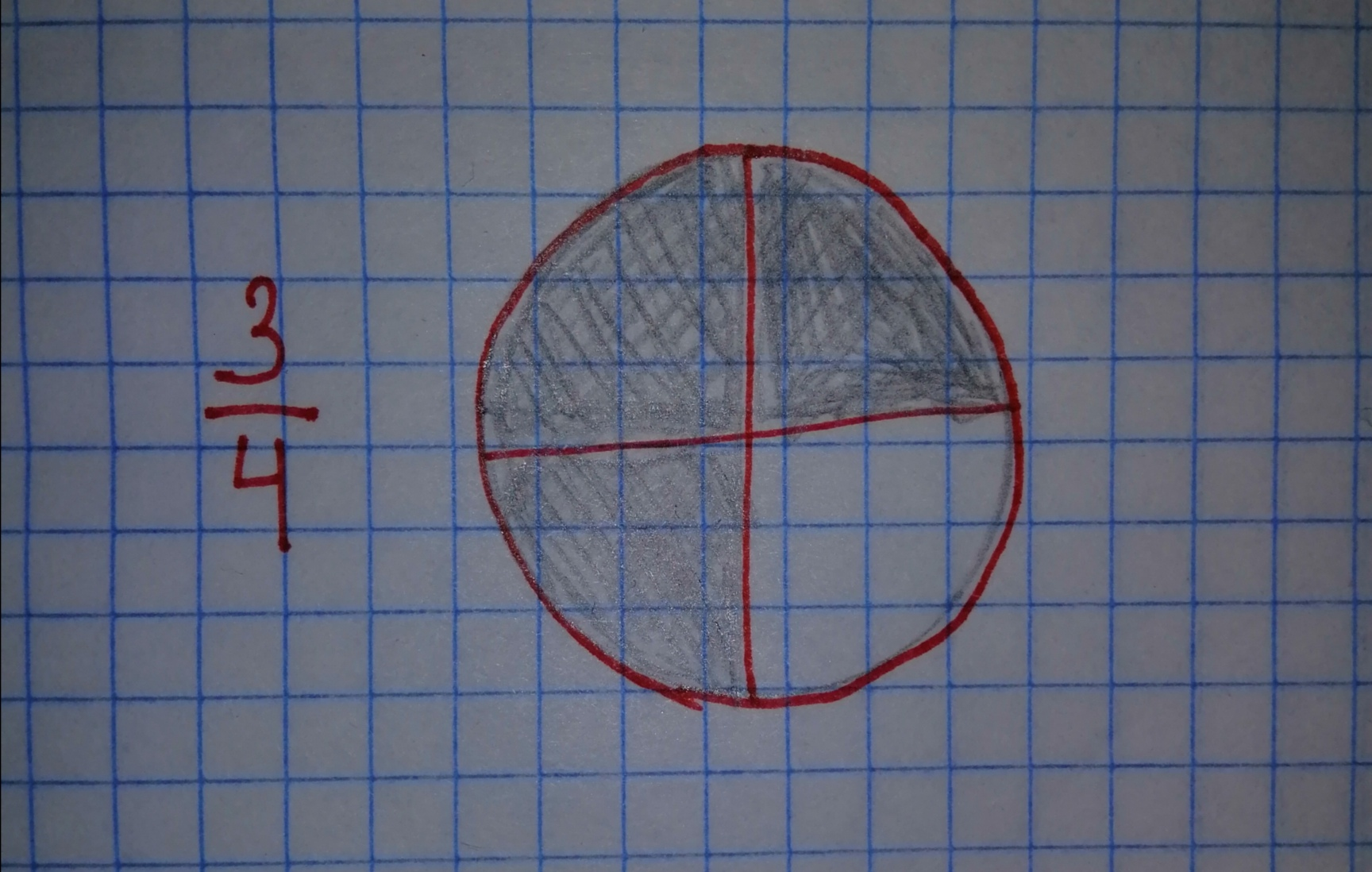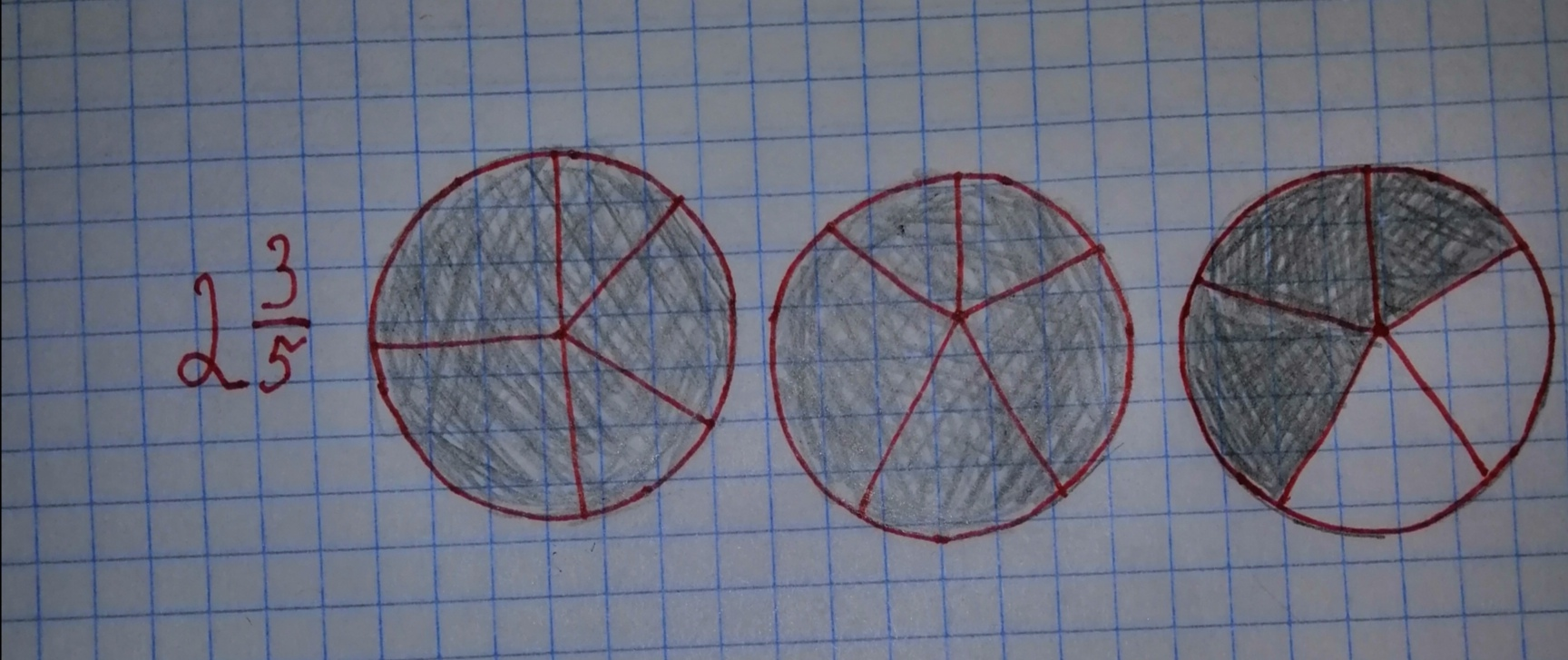# Modeling: Draw models to represents frac{3}{4} and 2frac{3}{5}.Cheyanne Leigh 2020-11-12 Answered
Modeling: Draw models to represents $\frac{3}{4}$ and $2\frac{3}{5}.$
You can still ask an expert for help

• Questions are typically answered in as fast as 30 minutes

Solve your problem for the price of one coffee

• Math expert for every subject
• Pay only if we can solve itMargot Mill

Step 1
The model for the fraction $\frac{3}{4}$ is shown below. You can think a circular pizza.
Now divide it 4 equal slices. Then $\frac{3}{4}$ represent three pizza slice.Step 2
The model for the fraction $2\frac{3}{5}$. First note that

You can think you have three circular pizzas. Now divide it each pizza by 5 equal slices. Then you have total slices. Then $2\frac{3}{5}$ represent total 13 pizza slices. That meas, two complete pizza and three slices from the third pizza.